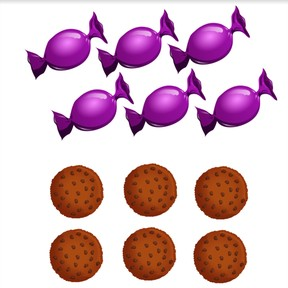Recognizing equal quantities 1 to 10

# Recognizing equal quantities 1 to 10

Recognizing equal quantities 1 to 10

No account needed.8,000 schools use Gynzy92,000 teachers use Gynzy1,600,000 students use Gynzy

## General

Students learn to recognize equal quantities from 1-10. They learn this by comparing different quantities with each other.

K.CC.C.6
K.CC.C.7

## Relevance

It is useful to know if you have more, less or equal quantities of something. An example is if you want to know if the chickens laid equal quantities of eggs.

## Introduction

Ask students to practice recognizing quantities from 1-10 in pairs. Give each pair 10 pencils. By turns students set down a random amount of pencils and the other student has to say the number. Finally check if students can recognize the different quantities of pencils. You can use the eraser or drag function to reveal the answer.

## Development

Put 9 glue sticks on one side of the table and 9 glue sticks on the other side. You can also choose to use another object. Are these in equal quantities? How do you know? First count one group out loud and then the other. Are the amounts the same? If so, they are an equal quantity. Practice this with an unequal quantity to show the difference. Check student's understanding by showing two images and asking them to compare. The students raise their hand if they are equal quantities.

To check that students understand equal quantities, you can ask the following questions:
What does equal quantities mean?
How do you know if something is in equal quantities?

## Guided Practice

Students practice by comparing different images with quantities from 1-10 and determining if they are in equal quantities or not.

## Closing

Check that students have understood by dragging an equal quantities of lollipops to the right side of the board. Students can also draw this individually. Ask students how they can check their answer. They can count both quantities and see if they are an equal quantity.

## Teaching Tip

Students who have difficulties should be encouraged to compare quantities to 5, and to practice counting to 10. Students who are ready to be challenged can be encouraged to compare numbers to 10.

## Instruction materials

Colored pencils, glue sticks

### The online teaching platform for interactive whiteboards and displays in schools

• Save time building lessons

• Manage the classroom more efficiently

• Increase student engagement